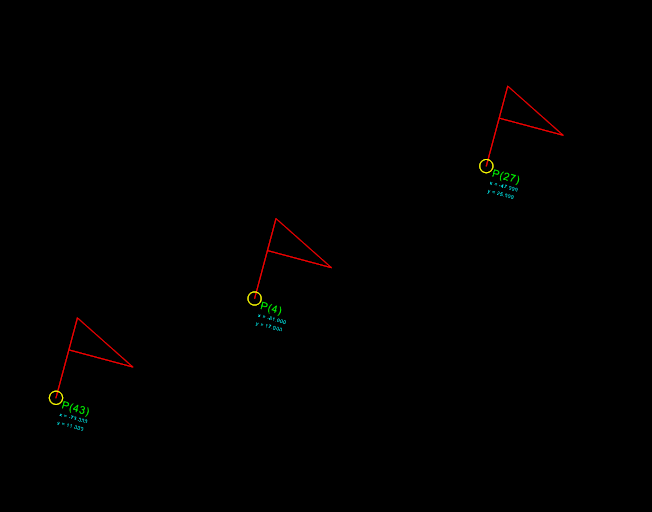# Insert2¶

Type: Composite Entity

Insert a new block-reference with auto-creating of ATTRIB from ATTDEF, and setting attrib-text by the attribs-dict.

`DXFEngine.``insert2`(blockdef, insert=(0., 0.), attribs={}, **kwargs)
Parameters: blockdef – the block definition itself insert – insert point (xy- or xyz-tuple), z-axis is 0 by default xscale (float) – x-scale factor, default=1. yscale (float) – y-scale factor, default=1. zscale (float) – z-scale factor, default=1. rotation (float) – rotation angle in degree, default=0. attribs (dict) – dict with tag:value pairs, to fill the the attdefs in the block-definition. example: {‘TAG1’: ‘TextOfTAG1’}, create and insert an attrib from an attdef (with tag-value == ‘TAG1’), and set text-value of the attrib to value ‘TextOfTAG1’. linetype (string) – linetype name, if not defined = BYLAYER layer (string) – layer name color (int) – range [1..255], 0 = BYBLOCK, 256 = BYLAYER

## Example¶

```import dxfwrite
from dxfwrite import DXFEngine as dxf

def get_random_point():
x = random.randint(-100, 100)
y = random.randint(-100, 100)
return (x, y)

sample_coords = [get_random_point() for x in range(50)]

flag_symbol = [(0,0), (0, 5), (4, 3), (0, 3)]

filename = 'flags.dxf'
dwg = dxf.drawing(filename)

# first create a block
flag = dxf.block(name='flag')
# add dxf entities to the block (the flag)
# use basepoint = (x, y) define an other basepoint than (0, 0)
# define some attributes
flag.add( dxf.attdef(insert=(0.5, -0.5), tag='NAME', height=0.5, color=3) )
flag.add( dxf.attdef(insert=(0.5, -1.0), tag='XPOS', height=0.25, color=4) )
flag.add( dxf.attdef(insert=(0.5, -1.5), tag='YPOS', height=0.25, color=4) )

# add block definition to the drawing
number = 1
for point in sample_coords:
# now insert flag symbols at coordinate 'point'
# insert2 needs the block definition object as parameter 'blockdef'
# see http://packages.python.org/dxfwrite/entities/insert2.html
# fill attribtes by creating a dict(), keystr is the 'tag' name of the
# attribute
values = {
'NAME': "P(%d)" % number,
'XPOS': "x = %.3f" % point,
'YPOS': "y = %.3f" % point
}
randomscale = 0.5 + random.random() * 2.0